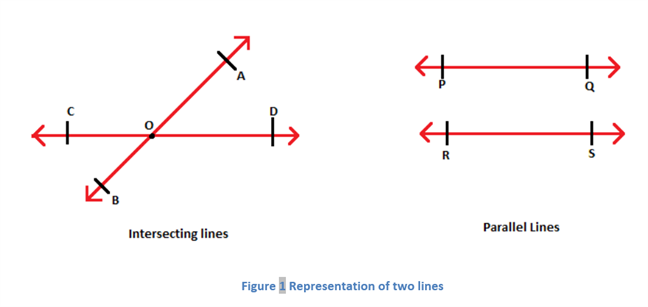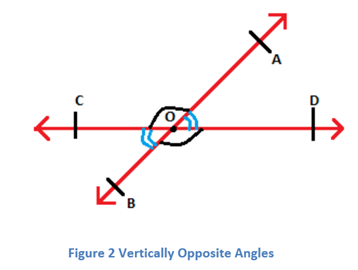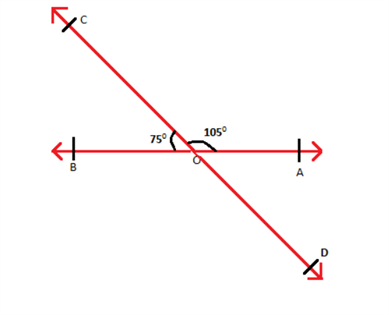# Vertical Angles (Vertically Opposite Angles)

When two lines intersect each other, then the opposite angles, formed due to intersection are called vertical angles or vertically opposite angles. A pair of vertically opposite angles are always equal to each other. Also, a vertical angle and its adjacent angle are supplementary angles, i.e., they add up to 180 degrees. For example, if two lines intersect and make an angle, say X=45°, then its opposite angle is also equal to 45°. And the angle adjacent to angle X will be equal to 180 – 45 = 135°.

When two lines meet at a point in a plane, they are known as intersecting lines. When the lines do not meet at any point in a plane, they are called parallel lines. Learn about Intersecting Lines And Non-intersecting Lines here.

## Definition

As we have discussed already in the introduction, the vertical angles are formed when two lines intersect each other at a point. After the intersection of two lines, there are a pair of two vertical angles, which are opposite to each other.

The given figure shows intersecting lines and parallel lines.In the figure given above, the line segment $$\overline{AB}$$ and $$\overline{CD}$$ meet at the point $$O$$ and these represent two intersecting lines. The line segment $$\overline{PQ}$$ and $$\overline{RS}$$ represent two parallel lines as they have no common intersection point in the given plane.

In a pair of intersecting lines, the angles which are opposite to each other form a pair of vertically opposite angles. In the figure given above, ∠AOD and ∠COB form a pair of vertically opposite angle and similarly ∠AOC and ∠BOD form such a pair. Therefore,

∠AOD = ∠COB

∠AOC = ∠BOD

For a pair of opposite angles the following theorem, known as vertical angle theorem holds true.

Note: A vertical angle and its adjacent angle is supplementary to each other. It means they add up to 180 degrees

## Vertical Angles: Theorem and Proof

Theorem: In a pair of intersecting lines the vertically opposite angles are equal.

Proof: Consider two lines $$\overleftrightarrow{AB}$$ and $$\overleftrightarrow{CD}$$ which intersect each other at $$O$$. The two pairs of vertical angles are:

i) ∠AOD and ∠COB

ii) ∠AOC and ∠BODIt can be seen that ray $$\overline{OA}$$ stands on the line $$\overleftrightarrow{CD}$$ and according to Linear Pair Axiom, if a ray stands on a line, then the adjacent angles form a linear pair of angles.

Therefore, ∠AOD + ∠AOC = 180° —(1) (Linear pair of angles)

Similarly, $$\overline{OC}$$ stands on the line $$\overleftrightarrow{AB}$$.

Therefore, ∠AOC + ∠BOC = 180° —(2) (Linear pair of angles)

From (1) and (2),

∠AOD + ∠AOC = ∠AOC + ∠BOC

⇒ ∠AOD = ∠BOC —(3)

Also, $$\overline{OD}$$ stands on the line $$\overleftrightarrow{AB}$$.

Therefore, ∠AOD + ∠BOD = 180° —(4) (Linear pair of angles)

From (1) and (4),

∠AOD + ∠AOC = ∠AOD + ∠BOD

⇒ ∠AOC = ∠BOD —(5)

Thus, the pair of opposite angles are equal.

Hence, proved.

### Solved Example

Consider the figure given below to understand this concept.In the given figure ∠AOC = ∠BOD and ∠COB = ∠AOD(Vertical Angles)

⇒ ∠BOD = 105° and ∠AOD = 75°

## Frequently Asked Questions – FAQs

### What is vertical angles?

When two lines intersect each other, then the angles opposite to each other are called vertical angles.

### How to measure vertical angles?

If the angle next to the vertical angle is given to us, then we can subtract it from 180 degrees to get the measure of vertical angle, because vertical angle and its adjacent angle are supplementary to each other.

### If x=30 degrees is a vertical angle, when two lines intersect, then find all the angles?

Given, vertical angle, x = 30
Let y is the angle vertically opposite to x, then y = 30 degrees
Now, as we know, vertical angle and its adjacent angle add up to 180 degrees, therefore,
The other two angles are: 180 – 30 = 150 degrees

### What are complementary angles with example?

The angles which are adjacent to each other and their sum is equal to 90 degrees, are called complementary angles. For example, x = 45 degrees, then its complement angle is: 90 – 45 = 45 degrees